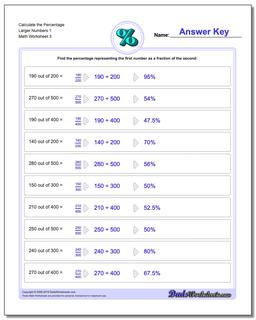# Math Worksheets: Percentages: Percentages: Calculate the Percentage Larger Numbers 1 (Third Worksheet)## Calculate the Percentage Larger Numbers 1 (Third Worksheet)

PropertyValue
DescriptionCalculate the Percentage Larger Numbers 1: Percent worksheets where students are given two numbers and must determine what percentage one number is of the other number. (Third Worksheet)
Resource TypeWorksheet